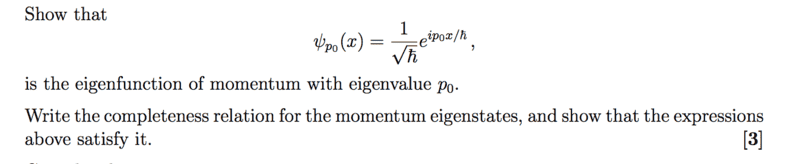# Eigenfunction of momentum and operators

## Homework Statement## Homework Equations

##\hat{P}= -ih d/dx##

## The Attempt at a Solution

To actually obtain ##\psi_{p_0}## I guess one can apply the momentum operator on the spatial wavefunction. If we consider a free particle (V=0) we can easily get obtain ##\psi = e^{\pm i kx}##, where ##k= \sqrt{2mE/ \hbar}##.

By now applying momentum operator we get ##\hat{P} \psi= -ih \cdot \pm i \sqrt{2mE/ \hbar} ##. This is as far as I get, how do I actually get the eigenfunction of momentum on the required form?

And 2:
How do I show that the completeness relation is satisfied for that 'specific' expression? I know that we can express ##|x> = \int dp |p><p|x>,##take ##-i \hbar \frac{d}{d_x} f_p(x) = p f_p(x)## and turn into a fourier transformation (including k in the kernel) and get ##p' \tilde{f_p(p')}## which can be solved picking p=p' and any function of p can be converted into an eigenfunction if we transform back to x s.t ##f_p(x) = \int dp e^{ipx \hbar} \tilde{f_p(p)}##, but I have no idea how to use this to confirm the completeness relation for the above expression....

#### Attachments

Orodruin
Staff Emeritus
Homework Helper
Gold Member
2021 Award
1) You do not need to derive the eigenfunction. Just show that the given function is the eigenfunction.

2) You need to show that ##\int |p\rangle \langle p| dp## is the identity operator, i.e., that acting on any state with it gives back the same state.

Ahh okey, that made the first part very easy.

2) You need to show that ∫|p⟩⟨p|dp∫|p⟩⟨p|dp\int |p\rangle \langle p| dp is the identity operator, i.e., that acting on any state with it gives back the same state.

And on 2) what exactly is p in that equation?

Orodruin
Staff Emeritus
Homework Helper
Gold Member
2021 Award
It is just a parameter labelling the momentum eigenstates.

It is just a parameter labelling the momentum eigenstates.
Okey, so I'm a bit confused about the state vs ##\psi_{p_0}## which is a function. Could I rewrite the function as a state somehow?

Okey, so I'm a bit confused about the state vs ##\psi_{p_0}## which is a function. Could I rewrite the function as a state somehow?
Because plugging in ##\psi## in the equation returns ##p_0^2 / \hbar \cdot \int dp. ##

Orodruin
Staff Emeritus
Homework Helper
Gold Member
2021 Award
The wave function ##\psi(x)## for any state ##|\psi\rangle## is defined as ##\psi(x) = \langle x|\psi\rangle##.

Oki I see. Are there way to get p for this specific case which I ultimately can plug into the completeness theorem?

Orodruin
Staff Emeritus
Homework Helper
Gold Member
2021 Award
There is no specific p. The ##p## in the completeness relation is an integration variable.

There is no specific p. The ##p## in the completeness relation is an integration variable.
If there's no specific, p, how can I check it for a specific case?

Orodruin
Staff Emeritus
Homework Helper
Gold Member
2021 Award
You should not be checking it for a specific case. You should show that it is always the identity operator.

You should not be checking it for a specific case. You should show that it is always the identity operator.
"Show that the expression above satisfies it"

Orodruin
Staff Emeritus
Homework Helper
Gold Member
2021 Award
"Show that the expression above satisfies it"
Yes? You are still not understanding the question correctly. The wavefunction you are given is the wavefunction corresponding to the state ##|p_0\rangle##. You need to show that the states with this type of wavefunction lead to the states satisfying the completeness relation.

Yes? You are still not understanding the question correctly. The wavefunction you are given is the wavefunction corresponding to the state ##|p_0\rangle##. You need to show that the states with this type of wavefunction lead to the states satisfying the completeness relation.
Cheers. Yes the reason I ask is that I obviously don't understand it.

Orodruin
Staff Emeritus
$$\langle x|p_0\rangle = \frac{1}{\sqrt{\hbar}} e^{ip_0x/\hbar}.$$
$$\int |x\rangle \langle x| dx = 1,$$
$$\langle x|\psi\rangle = \int \langle x|p \rangle \langle p |\psi \rangle dp$$